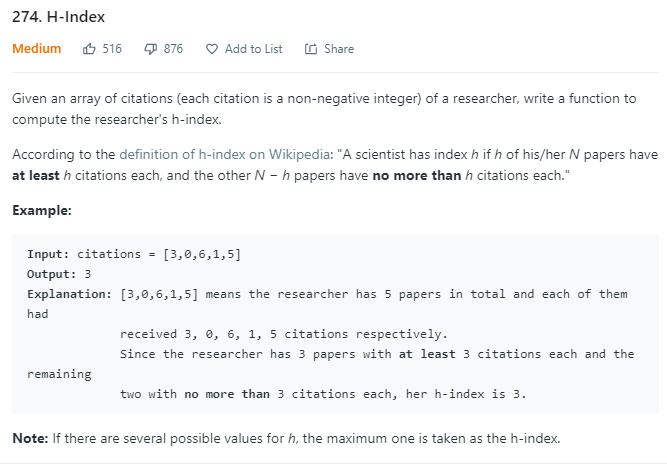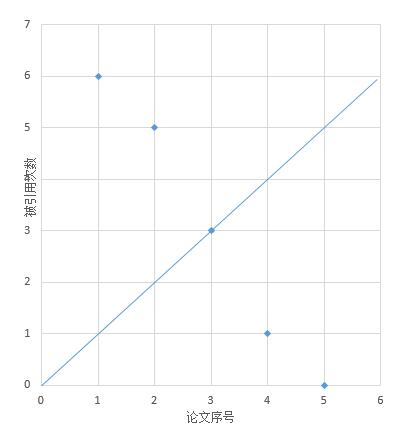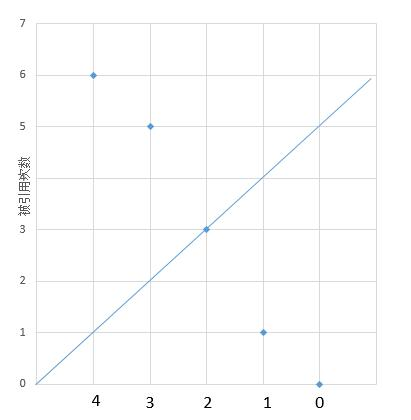# 题目描述（中等难度）H 指数，H 指数等于 n，代表该作者所发表的所有论文中至少有 n 篇论文的被引用次数大于等于 n

# 解法一

H指数的计算基于其研究者的论文数量及其论文被引用的次数。赫希认为：一个人在其所有学术文章中有N篇论文分别被引用了至少N次，他的H指数就是N。如美国耶鲁大学免疫学家理查德·弗来沃发表的900篇文章中，有107篇被引用了107次以上，他的H指数是107。

1. 将其发表的所有SCI论文按被引次数从高到低排序；
2. 从前往后查找排序后的列表，直到某篇论文的序号大于该论文被引次数。所得序号减一即为H指数。

public int hIndex(int[] citations) {
Arrays.sort(citations); // 默认的是从小到大排序，所以后边要倒着遍历
int n = 1; // 论文序号
//倒着遍历就是从大到小遍历了
for (int i = citations.length - 1; i >= 0; i--) {
// 论文序号大于该论文的被引次数
if (n > citations[i]) {
break;
}
n++;
}
// 所得序号减一即为 H 指数。
return n - 1;
}public int hIndex(int[] citations) {
Arrays.sort(citations);
int n = citations.length;
for (int i = 0; i < n; i++) {
// 点在直线上方
if (citations[i] >= n - i) {
return n - i;
}
}
return 0;
}


# 解法二

... ...

public int hIndex(int[] citations) {
int n = citations.length;
int[] buckets = new int[n+1];
//计数
for(int c : citations) {
if(c >= n) {
buckets[n]++;
} else {
buckets[c]++;
}
}
int count = 0;
//依次判断被引次数大于等于 N 的论文数是否大于等于 N
for(int i = n; i >= 0; i--) {
count += buckets[i];
if(count >= i) {
return i;
}
}
return 0;
}


public int hIndex(int[] citations) {
int n = citations.length;
int N = 0; // 记录引用数大于等于 n 的论文数
for (int i = 0; i < n; i++) {
int count = citations[i];
//已经记录过这个数
if (count < 0) {
continue;
}
//初始化为 0
citations[i] = -1; // -1 -> 0
//大于等于 n 的情况，用 N 统计
if (count >= n) {
N++;
continue;
}
//当前值之前是否被统计过
while (citations[count] >= 0) {
//保存当前论文被引用次数
int temp = citations[count];
//统计当前数，初始化为 1
citations[count] = -2; // -2 -> 1
count = temp;
//大于等于 n 的情况
if (count >= n) {
N++;
break;
}
}
//当前值之前已经被统计过，在原来的基础上减一(也就是计数加 1)
if (count < n && citations[count] < 0) {
citations[count]--;
}
}

// 全部论文引用数大于等于 n
if (N == n) {
return n;
}
int count = N;
for (int i = n - 1; i >= 0; i--) {
count = count + (~citations[i]);
if (count >= i) {
return i;
}
}
return 0;
}